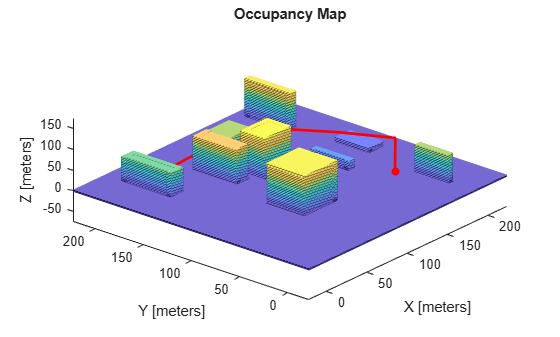# validatorOccupancyMap3D

State validator based on 3-D grid map

## Description

The `validatorOccupancyMap3D` object validates states and discretized motions based on occupancy values in a 3-D occupancy map. The object interprets obstacle-free map locations as valid states. The object interprets occupied and unknown map locations as invalid states.

## Creation

### Syntax

``validator = validatorOccupancyMap3D``
``validator = validatorOccupancyMap3D(stateSpace)``
``validator = validatorOccupancyMap3D(stateSpace,Name,Value)``

### Description

````validator = validatorOccupancyMap3D` creates a 3-D occupancy map validator associated with an SE(3) state space with default settings.```

example

````validator = validatorOccupancyMap3D(stateSpace)` creates a validator in the specified state space. The `stateSpace` input sets the value of the StateSpace property.```
````validator = validatorOccupancyMap3D(stateSpace,Name,Value)` sets Properties using one or more name-value pairs. Unspecified properties have default values. Enclose each property name in quotes.For example, `validatorOccupancyMap3D('ValidationDistance',0.1)` creates a 3-D occupancy map validator with a sampling interval of 0.1.```

## Properties

expand all

State space for validating states, specified as a subclass of `nav.StateSpace`. These are the predefined state space objects:

Example: `validatorOccupancyMap3D(stateSpaceSE3)`

Map used for validating states, specified as an `occupancyMap3D` object.

Example: `validator.Map = occupancyMap3D(10)`

Interval for sampling between states and checking state validity, specified as a positive numeric scalar.

Example: `validator.ValidationDistance = 0.1`

Data Types: `double`

State variable mapping for xyz-coordinates in the state vector, specified as a three-element vector of form `[xIdx yIdx zIdx]`.

Data Types: `double`

## Object Functions

 `copy` Create deep copy of state validator object `isMotionValid` Check if path between states is valid `isStateValid` Check if state is valid

## Examples

collapse all

Create a 3-D occupancy map and associated state validator. Plan, validate, and visualize a path through the occupancy map.

Load and Assign Map to State Validator

Load a 3-D occupancy map of a city block into the workspace. Specify a threshold for which cells to consider as obstacle-free.

```mapData = load('dMapCityBlock.mat'); omap = mapData.omap; omap.FreeThreshold = 0.5;```

Inflate the occupancy map to add a buffer zone for safe operation around the obstacles.

`inflate(omap,1)`

Create an SE(3) state space object with bounds for state variables.

```ss = stateSpaceSE3([-20 220; -20 220; -10 100; inf inf; inf inf; inf inf; inf inf]);```

Create a 3-D occupancy map state validator using the created state space.

`sv = validatorOccupancyMap3D(ss);`

Assign the occupancy map to the state validator object. Specify the sampling distance interval.

```sv.Map = omap; sv.ValidationDistance = 0.1;```

Plan and Visualize Path

Create a path planner with increased maximum connection distance. Reduce the maximum number of iterations.

```planner = plannerRRT(ss,sv); planner.MaxConnectionDistance = 50; planner.MaxIterations = 1000;```

Create a user-defined evaluation function for determining whether the path reaches the goal. Specify the probability of choosing the goal state during sampling.

```planner.GoalReachedFcn = @(~,x,y)(norm(x(1:3)-y(1:3))<5); planner.GoalBias = 0.1;```

Set the start and goal states.

```start = [40 180 25 0.7 0.2 0 0.1]; goal = [150 33 35 0.3 0 0.1 0.6];```

Plan a path using the specified start, goal, and planner.

`[pthObj,solnInfo] = plan(planner,start,goal);`

Check that the points of the path are valid states.

`isValid = isStateValid(sv,pthObj.States)`
```isValid = 7x1 logical array 1 1 1 1 1 1 1 ```

Check that the motion between each sequential path state is valid.

```isPathValid = zeros(size(pthObj.States,1)-1,1,'logical'); for i = 1:size(pthObj.States,1)-1 [isPathValid(i),~] = isMotionValid(sv,pthObj.States(i,:),... pthObj.States(i+1,:)); end isPathValid```
```isPathValid = 6x1 logical array 1 1 1 1 1 1 ```

Visualize the results.

```show(omap) hold on scatter3(start(1,1),start(1,2),start(1,3),'g','filled') % draw start state scatter3(goal(1,1),goal(1,2),goal(1,3),'r','filled') % draw goal state plot3(pthObj.States(:,1),pthObj.States(:,2),pthObj.States(:,3),... 'r-','LineWidth',2) % draw path```## Version History

Introduced in R2020b